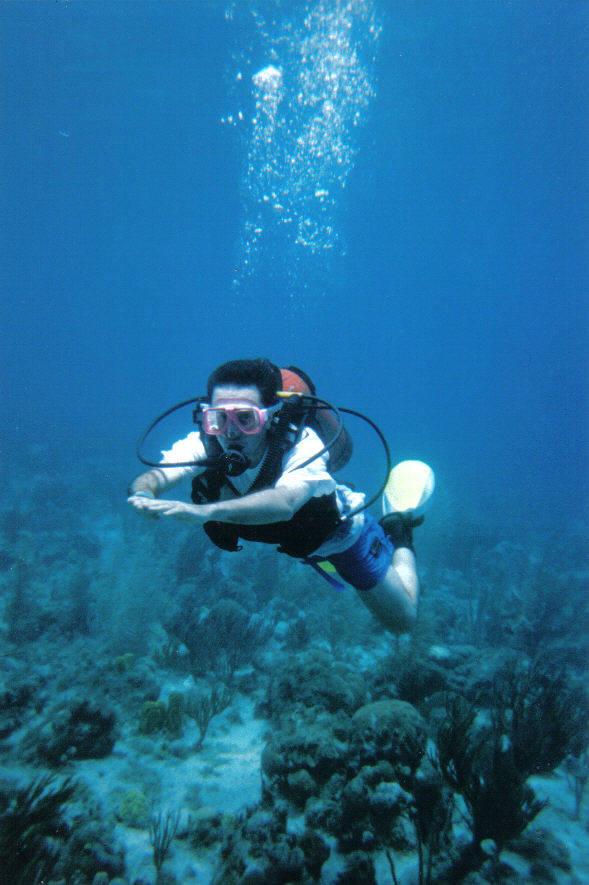## Buoyancy

If you were to ask a second grader, they might tell you that gravity is down and buoyancy is up.  For a long time, my son thought gravity pulled his bicycle down the hill and buoyancy was responsible for it to go up the hill.  Not quite.

But what is buoyancy anyways?  A textbook definition might be that the force of buoyancy is equal to the weight of the displaced fluid.  A way to think of this is that classically, we cannot have two objects occupy the same space.  Do if an objects sinks into a fluid (liquid OR gas) then it is taking up space and that fluid is pushed up. This is known as Archimedes Principle.

That displaced fluid wants to be as close to the ground as it can get.  The fluid wants to be in a state of lower gravitational potential energy.  The only way for the fluid to be lower is to push that invasive object out of the way, or UP!  Thus we have the force of buoyancy.

Your text might present the equation for buoyancy as

F = ρgV

Now there is some lack of clarity as to what these variable represent.  To be correct, ρ is the density of the displaced fluid, g is the acceleration due to gravity, and V is the volume of the displaced fluid. But to clarify, we need to know if the object is floating on the top of the surface of the fluid or completely submerged.

If the object is 100% submerged, then we can assume the volume of the displaced fluid is equal to the volume of the object itself.  This works nice whether or not the net force on the 100% submerged object is zero or not.

However, if the object is floating on the surface, the volume of the objects is NOT equal to the volume of the displaced fluid.  In this case, a neat trick we can use is to assume the net force equals zero (otherwise it would be sinking, not floating).  Thus the force of buoyancy is equal to the weight of the object.

F = mg

Where m is the mass of the object.   Now using our equation for density where

ρ = m/V,

we can equate the mass of the object equal to the density of the object times the volume

m=ρV

Substituting this in, we actually get the force of gravity (and thus the buoyancy) depending on the density and volume of the object, even if the equation is deceptively similar.

F = ρgV

Some things to consider is how could you experimentally measure the force of buoyancy on an object in water?

Where do you experience the buoyant force if you want to describe this to your little brother or sister?

What happens to the buoyant force on a SCUBA diver as the descend and ascend?Brave Physics Teacher Learning to SCUBA Dive in San Andreas, Colombia

You can play around with this PHET simulation to gain some experience with buoyancy.

…..